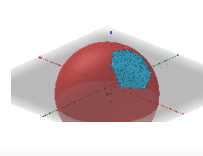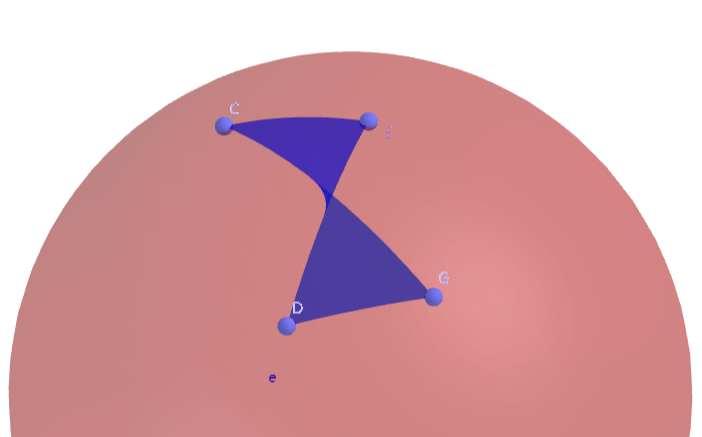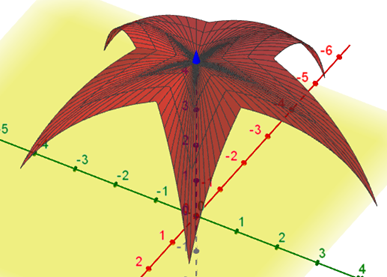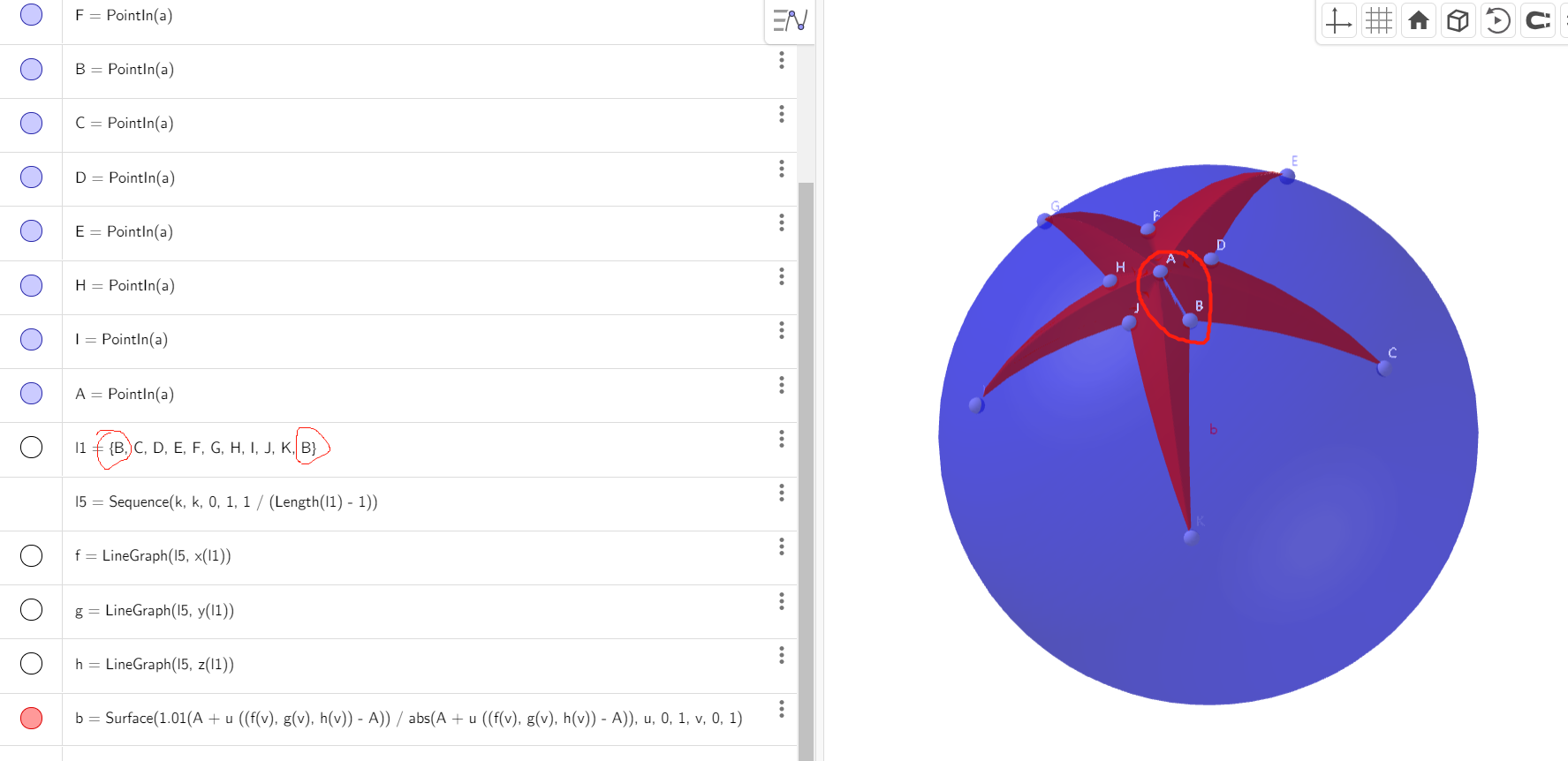# How to color a spherical triangle?

Nicholasfox shared this question 2 years ago

In the attachment, how to color the Spherical triangle ABC?creo que ahora funciona mucho mejorhttps://www.geogebra.org/m/nzds5za521

What a complex expression. Thank you very much.3

expresion is simple (1-u) (1-v) A+(1-u) v B+u (1-v) C +u v D with 0<=u<=1 0<=v<=1 is surface with A,B,C,D vertexes

when C=D is a triangle

the expression divided by its modulus is a spheric triangle

sums and differences of the center selects the adequate position1

I've since come to understand what it means.

1. I think it might be possible to use the UnitVector command, but didn't work. Can you please explain why?

Surface(A + R UnitVector((1 - u) (1 - v) (G - A) + (1 - u) v (C - A) + (1 - v) u (D - A) + v u (E - A)), u, 0, 1, v, 0, 1)

2. The result is related to the relative positions of the four points. I've tried convexhull command but it returns a locus, I can't get and sort the four points. Is there a way?Thank you very much.2

unitvector is a command not a function. Surface admits only functions. ie: max(f(x),g(x)) does not work, use (f+g+abs(f-g))/2 instead. Similar unitvector does not work use vector/abs(vector) instead

reordering the four points you can get good quadrilateral like in R2. move G to the left of C,D

use 1.01R for better view

Files: foro.ggb2

As for the UnitVector approach, I guess the problem is that UnitVector returns a vector object instead of a point object. It seems like there should be a simple way to convert a vector to a point, but I don't know how. Maybe then it would work. That said, I am even somewhat surprised that the Surface command accepts a point as the first argument. The manual only states Surface(<Expression>, <Expression>, <Expression>, ...) but not Surface(<Point>, ...), so maybe there is some automatic conversion.

As for your ConvexHull problem, you can get the ordered list of points via First(<Locus>, Length(<Locus>)-1), but there is a general problem with this approach. ConvexHull seems to return only a 2D locus, the convex hull of the projection of the points into the x-y-plane. Which means that not only would you have to match those points to the 3D version again (which would be doable though) but the result would often not be the one you want as you wouldn't necessarily get the "spherical convex hull" this way.

Btw, I assuming that you actually want a "spherical convex hull", i.e., it would become a triangle if one point lies within that "spherical convex hull". (Otherwise it'd not be quite clear which of the possible quadrangles would be the "correct" one.)1

artydent, Thanks.1

@ artydent

I did not follow the full plot, but multiply a vector by indentity makes a point - as long as it lasts1

Good to know, thanks. I am still somewhat confused sometimes about how points/vectors/matrices work in GeoGebra and when there is some automatic conversion and when not.

In this particular case it was a different problem though why it didn't work.1

Hi, mathmagic,

Is it feasible to generate a spherical pentagon(5 points) with an expression similar to this one?

(1-u) (1-v) A+(1-u) v B+u (1-v) C +u v D with 0<=u<=1 0<=v<=111

newer, shorter, nicer method in attached for surprising even more to Artydent

Files: foro.ggb1

This vector method is beyond my comprehension. But amazing.

Thanks.1

Very nice.

It's a base transformation (matrix m1), transforming the unit vectors onto the vectors AC, AD, AE, which means that the spherical triangle is the (shifted to center A) transformation of the octant of the unit sphere (surface b) which can be expressed nicely with spherical coordinates.1

posible es, pero no es realmente útil

Files: foro.ggb1

Maybe my computer's configuration is too low to display properly.

But it's much smoother after I set surface d to invisible.

I never thought it would be possible to express this surface in terms of parametric equations, This is really amazing.

Thank you for showing me the beauty of mathematics and GGB.1

creo que ahora funciona mucho mejorhttps://www.geogebra.org/m/nzds5za51

Definitely the best one.

Thanks.1

After a few hours of study, I finally understood your work. It's beautiful. Thanks.1

now it seems easy1

I used your method to make a similar one, but with a gap.1

GG crea una reticula de puntos en funcion de los parametros u y v

algunas veces la retícula va desde 0 hasta 0.99 ie. Esto significa que se ve una fina línea porque no se alcanza el recorrido al 100%

esto se remedia poniendo un valor del parámetro ligeramente superior al teórico. ie:

b = Surface(1.01(A + u ((f(v), g(v), h(v)) - A)) / abs(A + u ((f(v), g(v), h(v)) - A)), u, 0, 1, v, 0, 1.01)1

Got it. Thank you!